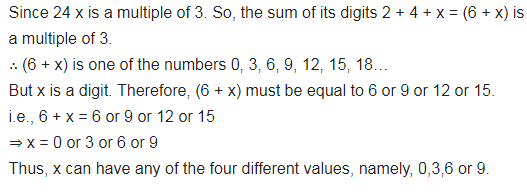# If 24x is a multiple of 3, where x is a digit, what is the value of x?

If 24x is a multiple of 3, where x is a digit, what is the value of x?